# License plate segmentation and recognition

5 days ago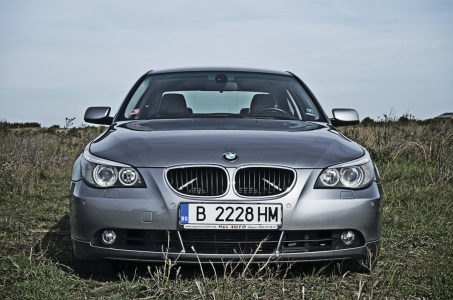When a computer vision project is approached you have to decide about what techniques to use, depending on the conditions of the project.

If the conditions of the problem are very controlled you can use a simple algorithm based on those restrictions (like using classical computer vision techniques to detect borders in the case of plate detection).

But, if the conditions of the problem are not easily controlled, very variant, messy, or even unknown, then you have to use techniques that can handle a lot of corner cases, which implies a more complex solution. This is the case of most real world applications.

That's when it makes sense to include a deep learning model, to be able to add more complexity in the logic of the solution, without defining that logic "manually" in the code. But this automation of the logic comes with the price of requiring data, lots of data in some cases: the more complex the logic, the bigger the amount of data needed. This data is used to automatically generate the logic using some machine learning model (for example: neural networks).

In this post, an example application of Computer Vision to license plates segmentation and recognition is used to show a possible way to approach a problem, providing a solution using a machine learning model. The algorithm is separated in two stages: license plate segmentation and license plate recognition.

You can find the code of this implementation in my Github repo.

The next image shows the general steps of the solution in the training and inference stages.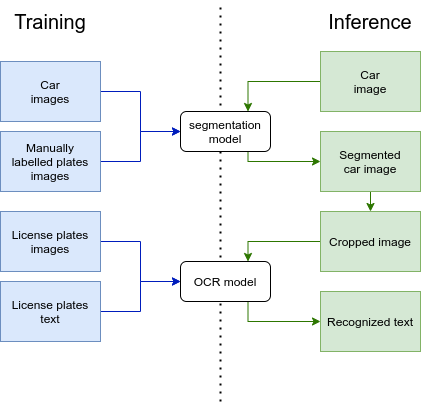In this stage a CNN (Convolutional Neural Network) is used as the model that performs the segmentation of the license plate in the image. This is done with the hope that the subsequent stage of recognition, focused on the license plate alone, could require less data, due to the reduced dimensionality of the input, for a given model complexity with a high precision. The model complexity (size of the model) is fixed to be able to limit the execution time of the inference.

More precisely, the CNN model corresponds to a UNet like model (UNet paper).

The model is trained with several car images and their manually generated segmentation masks.

Using the trained model, and an input car image the output segmentation mask of the model is shown below: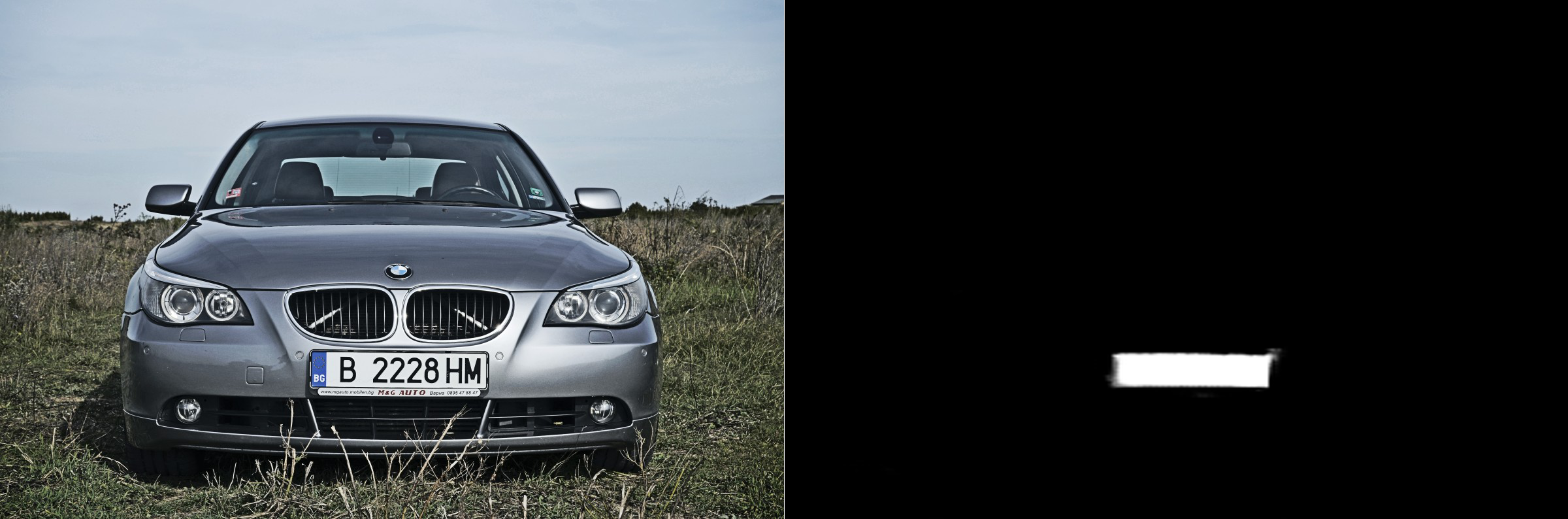Using the segmentation mask generated by the model, the plate can be highlighted: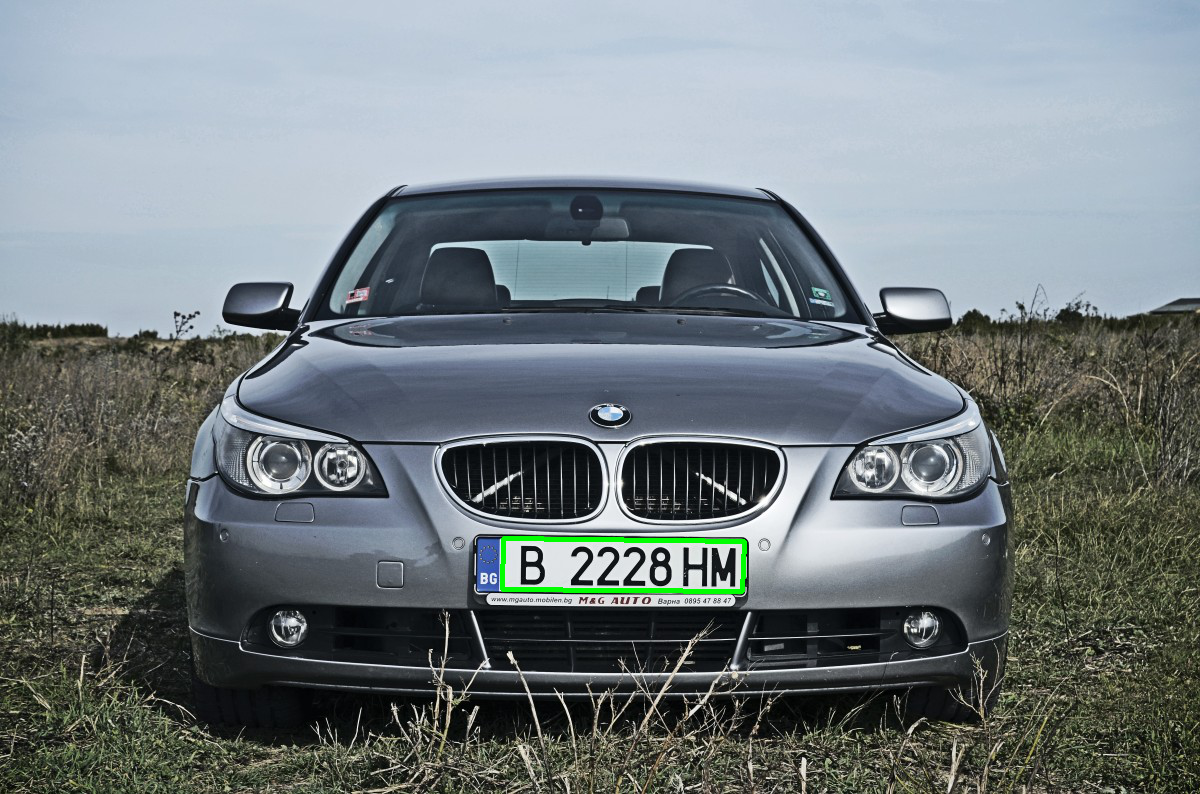And can be cropped and warped to get a rectangle containing the plate alone: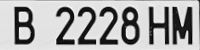Then, the trained segmentation model is used to crop all the training images. The cropped images and the corresponding manually labeled text is used to train a custom OCR model, to get the text (numbers and letters) of the license plate for each input image.

The model used for the recognition of the text of the license plate consists of several convolutional layers and some dense (fully connected) layers at the end. The output is a matrix with a fixed sized of M×N, where:

• M is the length of the amount of possible characters in the license plate (for example, the length of [A..Z1...9] would be 37).

• N is maximum possible length of the license plate text.

The process is illustrated in the next image: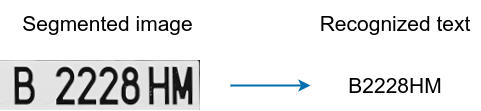## End to end solution

Using both trained models, is possible to generate the whole end to end process. So, for an input image, the segmented plate is highlighted and the recognized text is shown and also saved to a .csv file.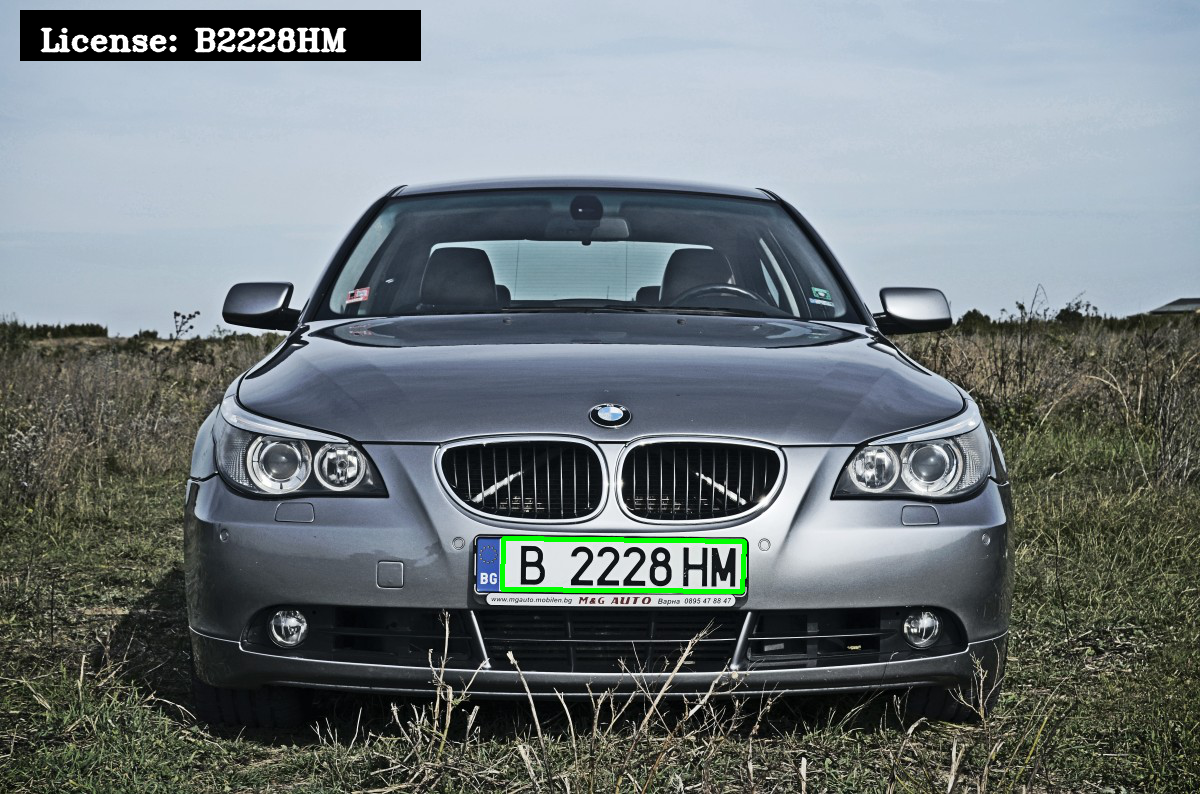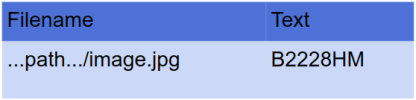You can find the code of this implementation in my Github repo.

Web developed in collaboration with

@jlasoc